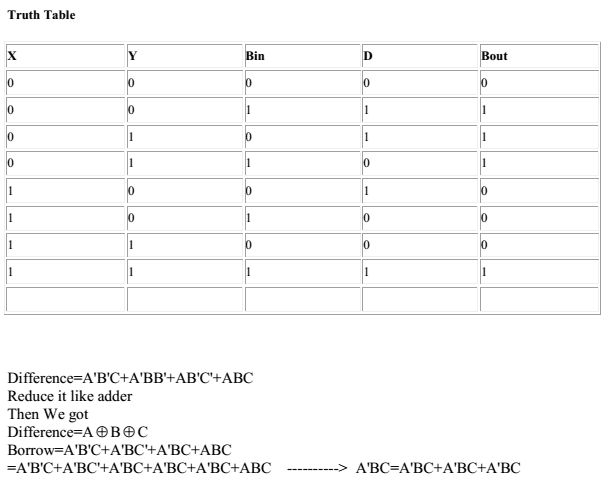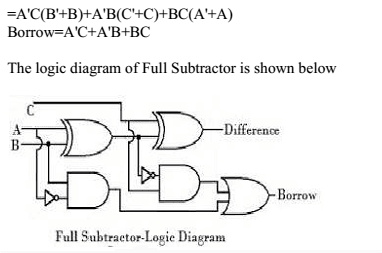# Arithmetic Circuits A+ A  A-

One of the most important tasks performed by a digital computer is the operation of adding
two binary numbers.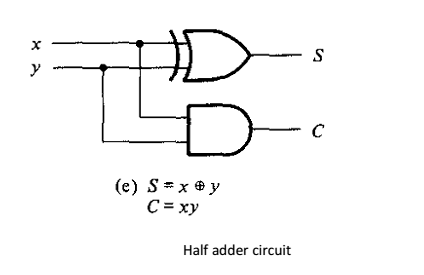The simplified Boolean functions for the two outputs can be obtained directly from the truth
table.

The simplified sum of products expressions are
S=x’y+xy’
C = xy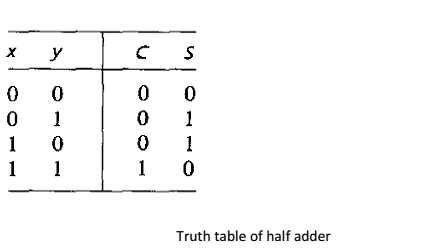A full-adder is a combinational circuit that forms the arithmetic sum of three input bits. It consists of three inputs and two outputs.
Two of the input variables, denoted by x and y, represent the two significant bits to be added. The third input, z, represents the carry from the previous lower significant position.The two outputs are designated by the symbols S for sum and C for carry. The binary variable S gives the value of the least significant bit of the sum. The binary variable C gives the output carry. The truth table of the full-adder is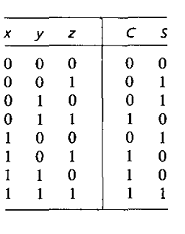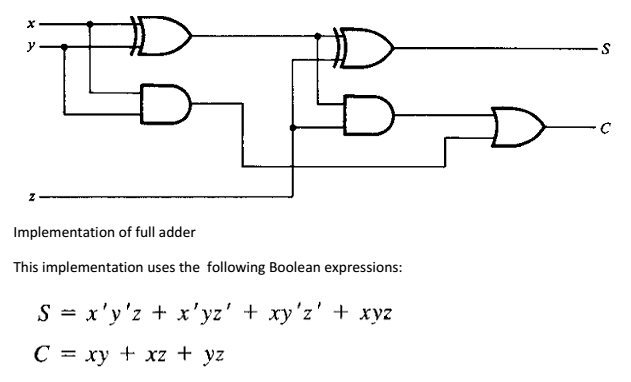Subtractor

Subtractor circuits take two binary numbers as input and subtract one binary number input from the other binary number input. Similar to adders, it gives out two outputs, difference and borrow (carry-in the case of Adder).

There are two types of subtractors.
• Half Subtractor
• Full Subtractor

Half Subtractor

The half-subtractor is a combinational circuit which is used to perform subtraction of two bits. It has two inputs, X (minuend) and Y (subtrahend) and two outputs D (difference) and B (borrow). The logic symbol and truth table are shown below.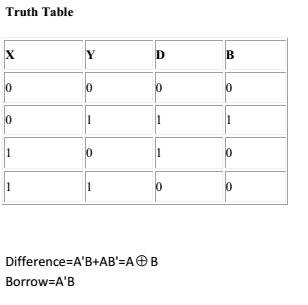The logic Diagram of Half Subtractor is shown below.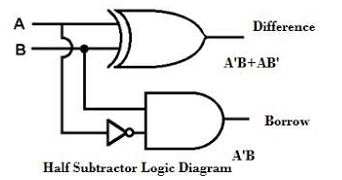Full Subtractor

A full subtractor is a combinational circuit that performs subtraction involving three bits, namely minuend, subtrahend, and borrow-in. so it allows cascading which results in the possibility of multi-bit subtraction. The truth table for a full subtractor is given below.